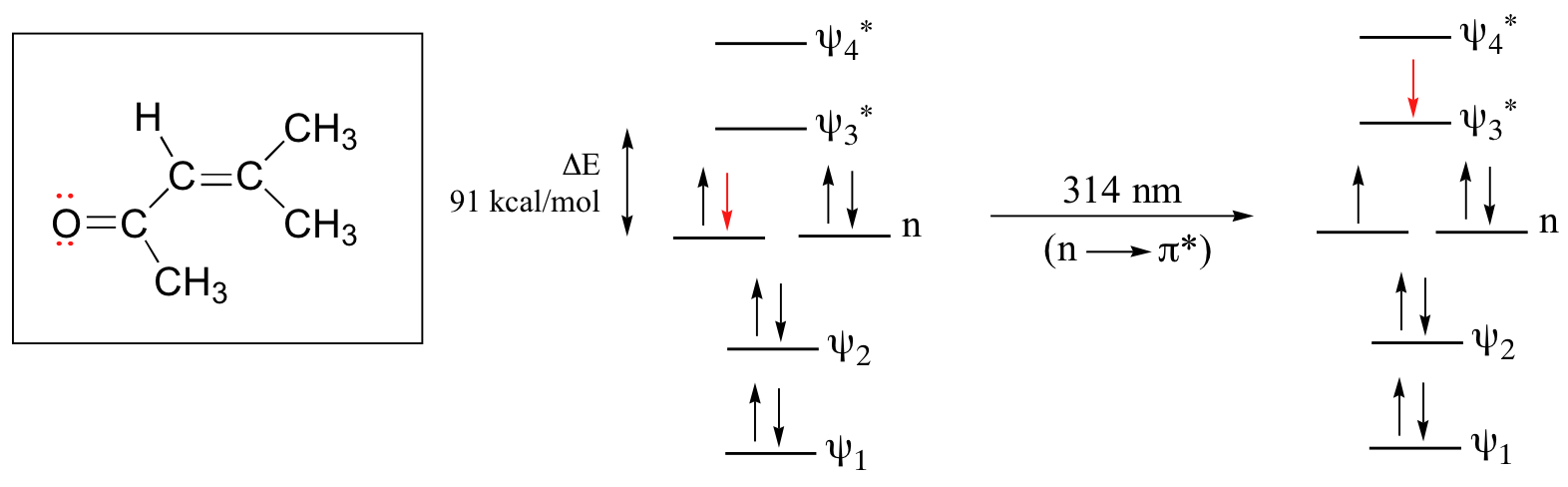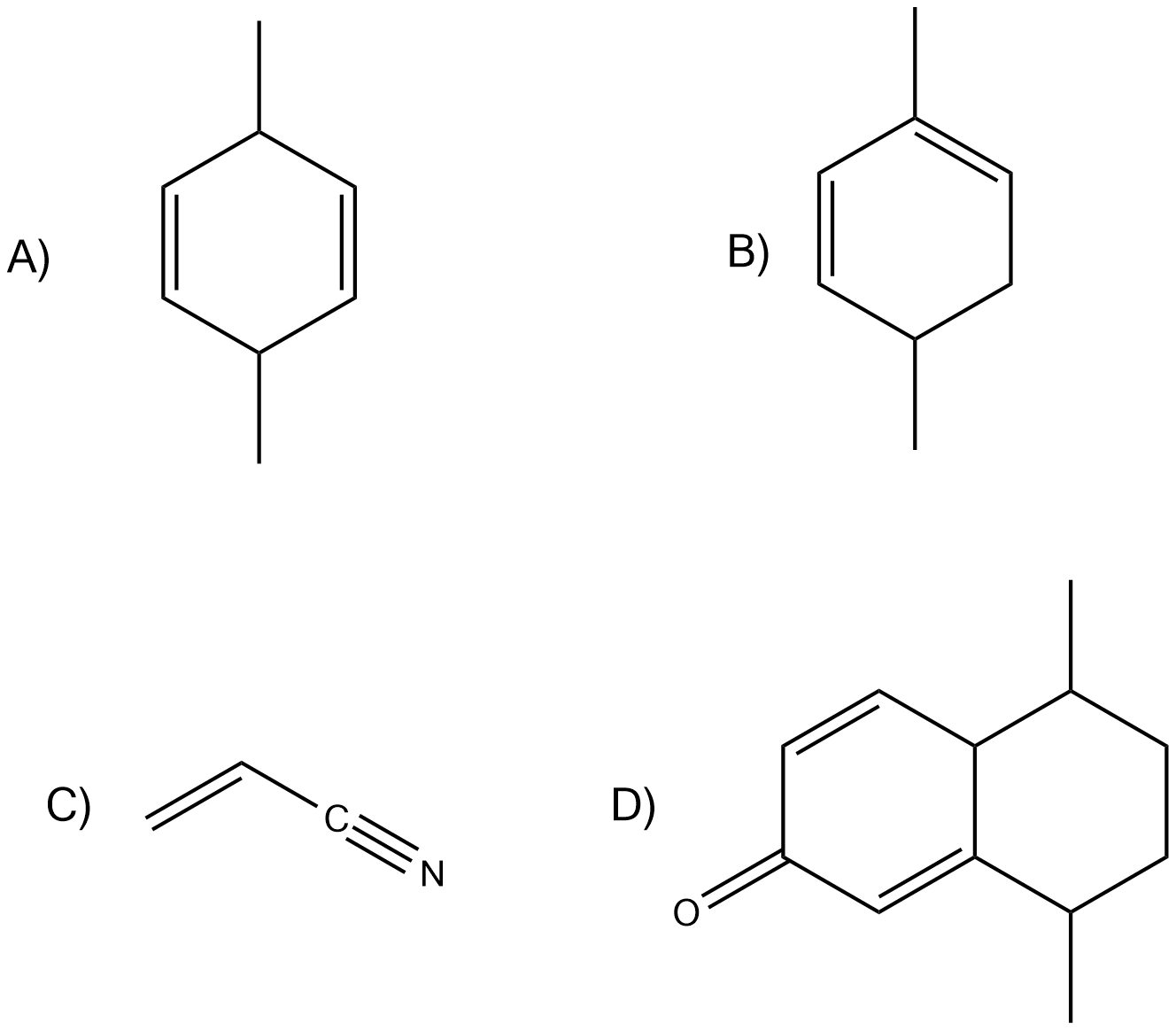## Interpreting UV Spectra

### Objectives

After completing this section, you should be able to use data from ultraviolet spectra to assist in the elucidation of unknown molecular structures.

### Study Notes

It is important that you recognize that the ultraviolet absorption maximum of a conjugated molecule is dependent upon the extent of conjugation in the molecule.

When a double-bonded molecule such as ethene (common name ethylene) absorbs light, it undergoes a ππ* transition.  Because π- π* energy gaps are narrower than σ σ* gaps, ethene absorbs light at 165 nm – a longer wavelength than molecular hydrogen.The electronic transitions of both molecular hydrogen and ethene are too energetic to be accurately recorded by standard UV spectrophotometers, which generally have a range of 220 – 700 nm.  Where UV-vis spectroscopy becomes useful to most organic and biological chemists is in the study of molecules with conjugated pi systems.  In these groups, the energy gap for π -π* transitions is smaller than for isolated double bonds, and thus the wavelength absorbed is longer.  Molecules or parts of molecules that absorb light strongly in the UV-vis region are called chromophores.

Let’s revisit the MO picture for 1,3-butadiene, the simplest conjugated system (see section 2.1B).  Recall that we can draw a diagram showing the four pi MO’s that result from combining the four 2pz atomic orbitals. The lower two orbitals are bonding, while the upper two are antibonding.Comparing this MO picture to that of ethene, our isolated pi-bond example, we see that the HOMO-LUMO energy gap is indeed smaller for the conjugated system. 1,3-butadiene absorbs UV light with a wavelength of 217 nm.

As conjugated pi systems become larger, the  energy gap for a π – π* transition becomes increasingly narrow, and the wavelength of light absorbed correspondingly becomes longer.   The absorbance due to the π – π* transition in 1,3,5-hexatriene, for example, occurs at 258 nm, corresponding to a ΔE of 111 kcal/mol.In molecules with extended pi systems, the HOMO-LUMO energy gap becomes so small that absorption occurs in the visible rather then the UV region of the electromagnetic spectrum.  Beta-carotene, with its system of 11 conjugated double bonds,  absorbs light with wavelengths in the blue region of the visible spectrum while allowing other visible wavelengths – mainly those in the red-yellow region – to be transmitted. This is why carrots are orange.The conjugated pi system in 4-methyl-3-penten-2-one gives rise to a strong UV absorbance at 236 nm due to a π – π* transition.  However, this molecule also absorbs at 314 nm.  This second absorbance is due to the transition of a non-bonding (lone pair) electron on the oxygen up to a π* antibonding MO:This is referred to as an n – π* transition.  The nonbonding (n) MO’s are higher in energy than the highest bonding p orbitals, so the energy gap for an n – π* transition is smaller that that of a π – π* transition – and thus the n – π* peak is at a longer wavelength. In general, n – π* transitions are weaker (less light absorbed) than those due to π – π* transitions.

### Looking at UV-vis spectra

We have been talking in general terms about how molecules absorb UV and visible light – now let’s look at some actual examples of data from a UV-vis absorbance spectrophotometer. The basic setup is the same as for IR spectroscopy: radiation with a range of wavelengths is directed through a sample of interest, and a detector records which wavelengths were absorbed and to what extent the absorption occurred.  Below is the absorbance spectrum of an important biological molecule called nicotinamide adenine dinucleotide, abbreviated NAD+. This compound absorbs light in the UV range due to the presence of conjugated pi-bonding systems.You’ll notice that this UV spectrum is much simpler than the IR spectra we saw earlier: this one has only one peak, although many molecules have more than one.  Notice also that the convention in  UV-vis spectroscopy is to show the  baseline at the bottom of the graph with the peaks pointing up.  Wavelength values on the x-axis are generally measured in nanometers (nm) rather than in cm-1 as is the convention in IR spectroscopy.

Peaks in UV spectra tend to be quite broad, often spanning well over 20 nm at half-maximal height.  Typically, there are two things that we look for and record from a UV-Vis spectrum..  The first is λmax, which is the wavelength at maximal light absorbance.  As you can see, NAD+ has λmax, = 260 nm.  We also want to record how much light is absorbed at λmax.  Here we use a unitless number called absorbance, abbreviated ‘A’.  This contains the same information as the ‘percent transmittance’ number used in IR spectroscopy, just expressed in slightly different terms.  To calculate absorbance at a given wavelength, the computer in the spectrophotometer simply takes the intensity of light at that wavelength before it passes through the sample (I0), divides this value by the intensity of the same wavelength after it passes through the sample (I), then takes the log10 of that number:

A = log I0/I

You can see that the absorbance value at 260 nm (A260) is about 1.0 in this spectrum.

### Example

Express A = 1.0 in terms of percent transmittance (%T, the unit usually used in IR spectroscopy (and sometimes in UV-vis as well).

### Solution

Here is the absorbance spectrum of the common food coloring Red #3:Here, we see that the extended system of conjugated pi bonds causes the molecule to absorb light in the visible range.  Because the  λmax of 524 nm falls within the green region of the spectrum, the compound appears red to our eyes.

Now, take a look at the spectrum of another food coloring, Blue #1:Here, maximum absorbance is at 630 nm, in the orange range of the visible spectrum, and the compound appears blue.

### Example 2

How large is the π – π* transition in 4-methyl-3-penten-2-one?

### Example 3

Which of the following molecules would you expect absorb at a longer wavelength in the UV region of the electromagnetic spectrum? Explain your answer.### Exercise

#### Question

Which of the following would show UV absorptions in the 200-300 nm range?